American Journal of Theoretical and Applied Statistics
Volume 5, Issue 5, September 2016, Pages: 260-269

Application of Loess Procedure in Modelling Geothermal Well Discharge Data from Menengai Geothermal Wells in Kenya

Madegwa James Etyang1, *, Edward Gachangi Njenga2

Department of Mathematics, Kenyatta University, Nairobi, Kenya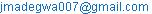(M. J. Etyang)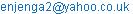(E. G. Njenga)

*Corresponding author

Madegwa James Etyang, Edward Gachangi Njenga. Application of Loess Procedure in Modelling Geothermal Well Discharge Data from Menengai Geothermal Wells in Kenya. American Journal of Theoretical and Applied Statistics. Vol. 5, No. 5, 2016, pp. 260-269. doi: 10.11648/j.ajtas.20160505.12

Received: July 15, 2016; Accepted: July 22, 2016; Published: August 6, 2016

Abstract: To measure the output of a geothermal well, also known as amount of megawatts of a well, discharge tests are done between two to four months after drilling of the well to collect the relevant types of data which includes wellhead pressure, lip pressure and the weir height. After collection of these data,  formula is applied in determining the well output. These data exhibits skewness and excess kurtosis also known as heavy – tailedness, an attempt to fit ordinary least squares (OLS) model to such data leads to model misspecification. Therefore, in this study, robust non-parametric estimation has been used to fit these data as applied by . The model is known to be robust to outliers which characterize the wells data, robustness signifies insensitivity to deviations from the strict model assumptions. A comparison between the robust method used and OLS method has also been made with graphical illustrations. The results show that locally weighted regression (loess) method used with a smoothing parameter of 0.07 and a polynomial of order 2 fits the geothermal well discharge data. It was confirmed that geothermal well discharge data is characterized by outliers which may affect the ultimate determination of the value of a well output and therefore there is need for further statistical data processing to remove the errors before Russel James method is applied.

Keywords: Locally Weighted Regression, Wellhead Pressure, Lip Pressure, Weir Height, Geothermal Well Output

1. Introduction

Geothermal exploration in Kenya dates back more than 40 years ago  where it was recognized that the central Rift Valley could contain a geothermal energy resource. In 1956 two wells were drilled with the second one X2 reaching a depth of 1035 meters. A detailed exploration of the geothermal resources in the Menengai area was conducted in 2004 and later with infill work in 2010. Geo-scientific investigations comprising geology, geophysics, geochemistry and heat loss measurements were utilized in searching for indicators for the existence of geothermal resources in the area . Findings presented in  and  point to the existence of exploitable geothermal resources within the Menengai caldera, Ol-Rongai and OlBanita calderas to the northwest of the Menengai caldera.  proceeds and concludes that the existence is evidenced by active strong surface manifestations and young lava's, signifying an active heat source.

Drilling activities in Menengai geothermal field begun in February 2011 . During drilling there are several tests that take place to determine reservoir properties. When well drilling is completed, a wellhead is fitted at the top of the well to control the steam as well as enable other relevant tests to be carried. This includes the discharge test which takes place between 2 to 4 months after completion of the drilling process. One of the basic tasks of a geothermal reservoir engineer is to measure the fluid flow from a discharging well and its energy content as well as to analyze the flow characteristics of the well. After 2 to 4 months heat up, the well is opened up and allowed to flow to the atmosphere. Geothermal high temperature wells are usually discharged into a silencer which also acts as a steam – water separator at atmospheric pressure .

There are several methods applied to determine a geothermal well output using the discharge data, of interest is the lip pressure method which was used in calculating the output of wells at the Menengai Caldera. The lip pressure method is based on an empirical formula developed by Russel James in 1962 .

To use the lip pressure method, the steam – water mixture is discharged through an approximately sized pipe into a silencer or some other simple device to separate the steam and water phases at atmospheric pressure . Water flow from the silencer is commonly measured by the weir – box method .  reiterated that well MW-01 was discharged in May, 2011. In determining the flow characteristics of the well, James lip pressure method was used. Discharge pipes of varying sizes; 8", 6", 5", 4" and 3" were used during discharge test. From the discharge tests, well-head pressure (WHP), lip pressure (PC) and the weir height data are collected which are used in estimation of the well output.

2. Literature Review

2.1. Non-parametric Regression

 denotes that there are generally two strategies for fitting a smooth curve which are parametric and non-parametric. The parametric methods require one to specify the functional form of the relationship in advance while non-parametric does not. He acknowledges that parametric fitting is a very effective way to summarize a relationship when the structure in the data is known but the challenge is that the "correct" structure of the data is almost always unknown. This causes researchers to risk fitting smooth curve that misrepresents the structure within data. Furthermore, he points out that non-parametric smoothing address this problem by locating smooth curve among data points without requiring any advance specification of functional relationship between variables.

2.2. Local Polynomial Regression

Local regression is actually an approach to fitting curves and surfaces to data by smoothing . It involves finding the value of parametric function to those observations in a neighborhood of a certain point of independent variables. Local polynomial regression are majorly used in providing an explanatory graphical tool as this gives insight into the behavior of the data and help in choosing parametric models, provide additional regression diagnostics to check the adequacy of parametric models fitted to the data and lastly to use locally weighted regression estimate as the estimated regression surface, without resorting to a parametric class of functions .

2.3. Regression Surface & Regression Errors

 states that although the locally weighted regression, also known as loess, procedure is non-parametric where functional form of the final smooth curve need not be specified, there are some parameters that must be selected prior to the fitting procedure. This guarantees that the loess curve passes through the center of the empirical data points. There are four items that must be selected in order to carry out locally weighted regression: the smoothing parameter (α), the degree of the polynomial (λ), the weight function and number of iterations. Out of these the most critical during local regression are the smoothing parameter and the order of the polynomial .

In locally weighted regression analysis, a decision has to be made on whether the data follows a Gaussian distribution or a symmetric distribution which depends on the type of error distribution; errors with tails that are stretched out leads to symmetric distribution while those that are independent random variables with mean 0 are termed to be normally distributed or having a Gaussian distribution. Errors with symmetric distribution lead to robust methods of estimation.

3. Findings and Discussion

3.1. Selection of Smoothing Parameter (α)

The smoothing parameter (α) also known as bandwidth, determines the width of the sliding window. It gives the proportion of observations that is to be used in each local regression .  explains that increasing α increases the smoothness of the smoothed points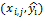. He further proposes that the goal in choosing of α is picking a value as large as possible to minimize the variability in the smoothed points without distorting the pattern in the data. That is, the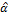should have as little bias as possible and a small variance as possible.  explain that there is need to strike a balance between bias and variance when selecting the α parameter.

Nearest neighbor smoothing parameter are widely used for local regression due to its flexibility in fitting most of the data. A fixed smoothing parameter estimate often has dramatic swings in variance due to large changes in the density of the data in the design space leading to unacceptably noisy fits .

 states that the α parameter is chosen as a value between 0 and 1. He further explains that the fitted curve becomes smoother with larger values of the smoothing parameter because wider fitting windows, larger α, means that idiosyncratic observations will tend to cancel each other out and therefore have proportionally less influence on the local regressions. Secondly, larger α values means that fewer observations will change when moving from one fitting window to the next. The above factors stabilize the local regression lines and fitted values, thereby producing a smoother curve.

The α parameter gives the proportion of observations to be used in each local regression. The smoothing parameter is specified as a value between 0 - 1 . α is supposed to be chosen on the basis of the characteristics of the data on the scatter plot. When choosing α, the goal should be to select a value as large as possible to minimize the variability in the smoothed points without distorting the pattern in the data . He further explains that selection of the smoothing parameter is a trade – off between bias and variance, that is the α should have as little bias as possible and a small variance as possible.

The results in Table 1 below show that the optimal smoothing parameter is 0.07 with the lowest standard error of 1.520978e-07 and bias of 7.176888e-06. Increasing the smoothing parameter leads to over smoothing while decreasing the smoothing parameter leads to under smoothing which is manifested by the increasing standard errors below and above the optimal smoothing parameter. It can be deduced from the figures above that, large smoothing parameters reduce the variance by smoothing over a large number of points, leading to increased bias. In contrast, small smoothing parameters give higher variance but have less bias hence lower bias leads to closer match between estimated values and actual observed values which can be shown by closer matching values of estimated megawatts (MWe) values with smoothing parameter of 0.07 and the true MWe values.

Figures 1, 2 and 3 below shows a graphical representation of actual megawatts values and estimated megawatts values for α=0.05, α=0.07 and α=0.09 respectively. For α= 0.05, a deviation from the actual values is seen by the blue line in the combined graph of Actual MWe & Estimated MWe. That is the estimated MWe values deviated from the 13th element to the 23rd element with the estimated values obtained as zero hence the high standard error. The same case is seen in Figure 3 for values ranging from 120 to 138. For a smoothing parameter of 0.07, the estimated values were the same as actual values thus the similarity in the graphs between the actual and estimated values. With the lowest standard deviation and bias, the optimal smoothing parameter was 0.07.

Table 1. Standard errors and variance for various smoothing parameter values.

 Smoothing Parameter Standard Error Bias 0.01 12.20789 -3.385072 0.02 3.519923 -1.134348 0.03 0.5870768 -0.2062319 0.04 0.3433087 -0.122174 0.05 0.3433087 -0.1221707 0.06 4.015572e-07 -8.365687e-06 0.07 1.520978e-07 7.176888e-06 0.08 1.622617e-06 -1.519279e-06 0.09 8.001929e-06 0.0001170377 0.10 1.398312e-05 -0.0001202426 0.15 4.586705e-05 -1.991862e-05 0.20 0.0003349328 0.0001960178 0.30 0.0005596501 0.000482664 0.35 0.0007297819 0.0003743899 0.40 0.0008338917 0.0006361917 0.50 0.001128271 0.001070689 0.75 0.001604839 0.0004933345 0.80 0.001694527 0.0003155935

3.2. Selection of Order of Polynomial (λ)

The α parameter specifies the degree/order of the polynomial that the local polynomial regression procedure fits to the data . The choice of the polynomial degree is a bias - variance trade off just like in the selection of the smoothing parameter. A higher degree will generally produce a less biased but more variable estimate than a lower degree one .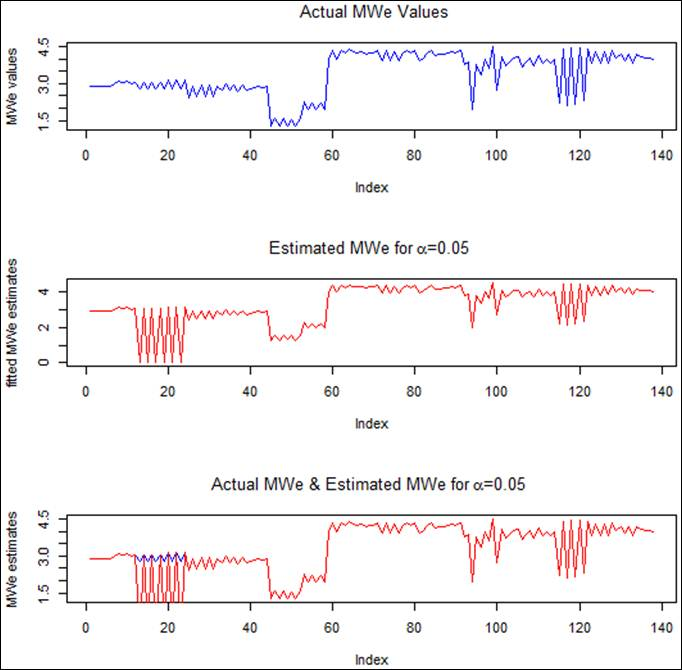Figure 1. Graph showing the fit of actual megawatts, estimated megawatts and a comparison between the two with λ = 2 and α = 0.05.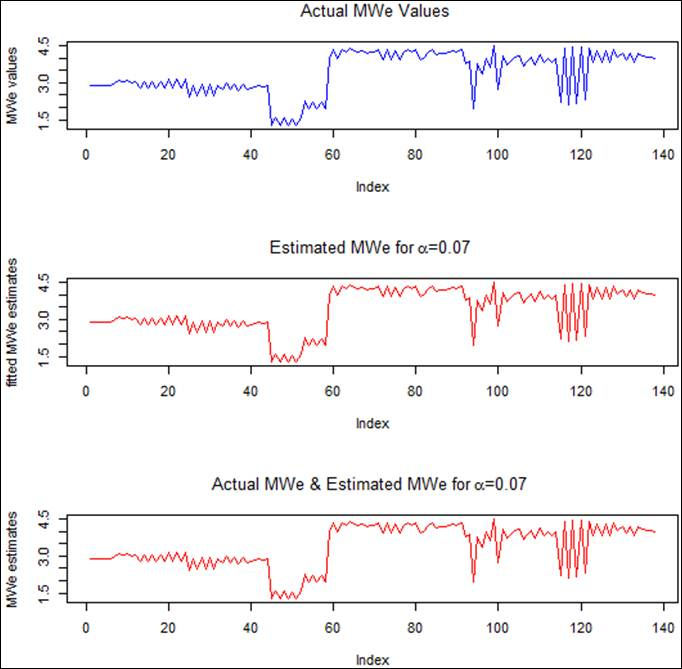Figure 2. Graph showing the fit of actual megawatts, estimated megawatts and a comparison between the two with λ = 2 and α= 0.07.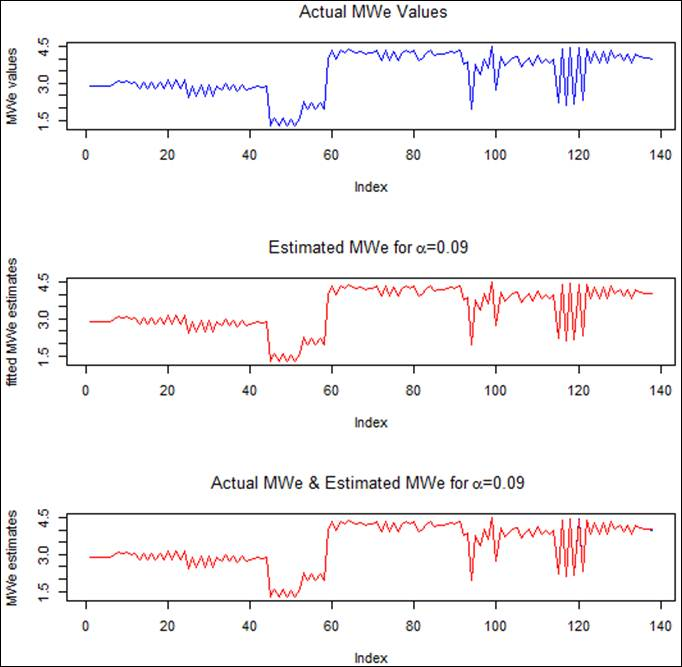Figure 3. Graph showing the fit of actual megawatts, estimated megawatts and a comparison between the two with λ = 2 and α= 0.09.

 states that the specification of the λ parameter is usually fairly easy because it can be decided by visual inspection of the scatter plot alone. He further explains that if the point cloud conforms to a generally monotonic pattern (either increasing or decreasing), then λ should be set to 1 for locally linear fitting and if the data exhibit some non-monotone pattern, with local minima and/ or maxima, then λ should be set to a value of 2 for locally quadratic equations. The reasons behind the above recommendations as explained by  is that if X and Y exhibits a monotonic relationship, then the point clouds within the local fitting windows should always exhibit the same general orientation. When this occurs, varying the intercepts and slopes of the locally linear regressions should be sufficient to produce a smooth curve that follows the data accurately. On the other hand, a non-monotonic relationship implies that the general orientation of the bi-variate point cloud changes direction somewhere within the data region of the scatter plot and as such reversals cannot be handled very effectively with linear equations. The quadratic specification allows for sharper inflections within the locally-fitted curve. This, in turn, produces the flexibility that is required to insure that the final local regression curve passes through the center of a non-monotone point cloud.

 explains that fitting polynomial with λ = 0 is the simplest because it is assumed that the equation if constant but it is better to assume local linearity. Choosing λ to be 1 appears to strike a good balance between computational ease and the need for flexibility to reproduce patterns in the data. With λ = 1 linear equations are fit within each of the windows. He finally denotes that for λ = 2 (where quadratic equations are used) computational consideration begin to override the need for flexibility.  puts forth that although λ = 2 complicates the fitting process, they are sometimes necessary in order to produce a smooth curve that follows the data to an acceptable degree.

Figure 4 below depicts that if we take λ = 0, with the other variables remaining the same, then we could not fit the data since the estimated values are different from the actual values as seen with the blue and red lines in the combined graph.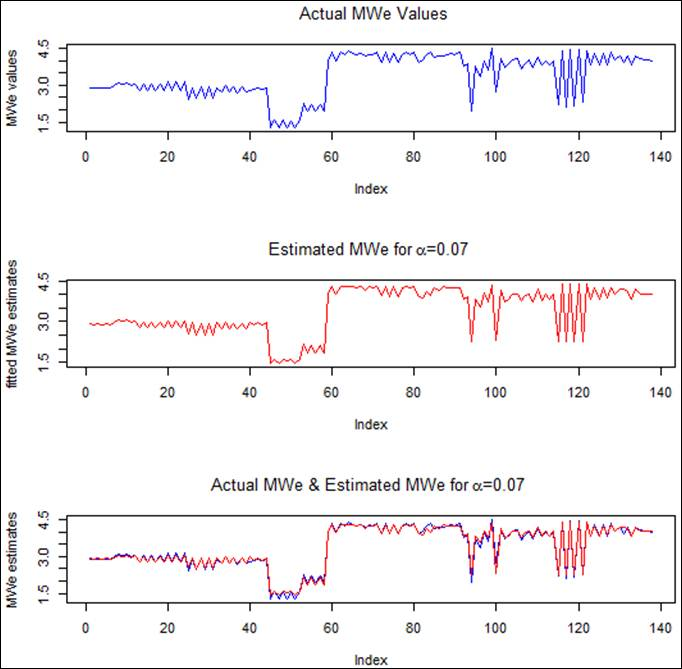Figure 4. Graph showing the fit of actual megawatts, estimated megawatts and a comparison between the two with α= 0.07 and λ = 0.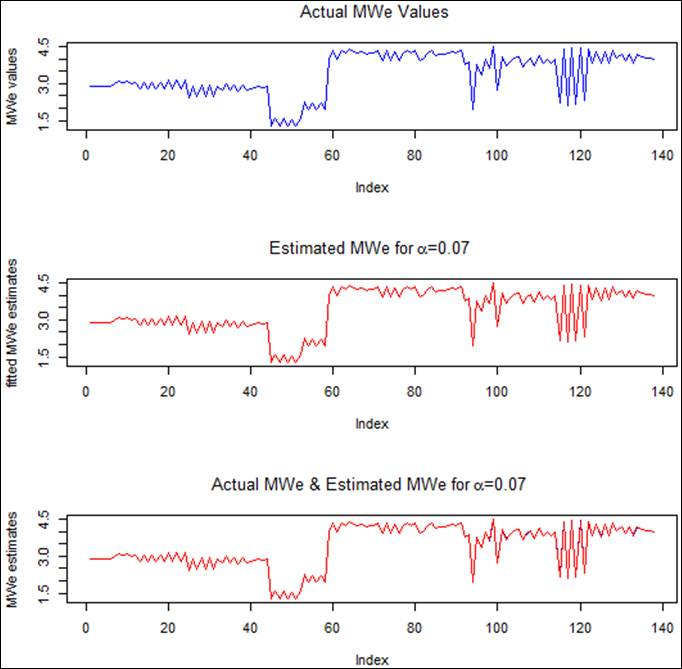Figure 5. Graph showing the fit of actual megawatts, estimated megawatts and a comparison between the two with α= 0.07 and λ = 1.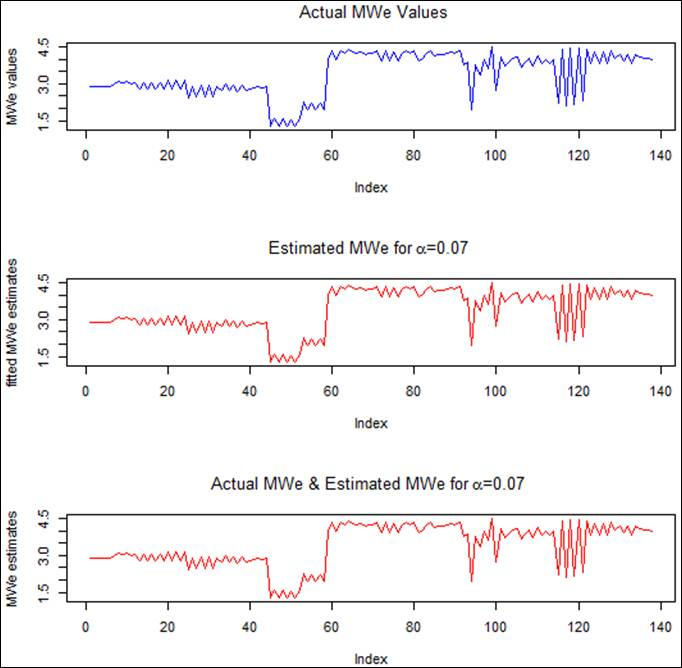Figure 6. Graph showing the fit of actual megawatts, estimated megawatts and a comparison between the two with α= 0.07 and λ = 1.

Figure 5 shows that as much as research has it that polynomial of degree 1 almost always provide adequate smoothed points, it is not the case. λ = 1 was not able to fit all data as blue points can be seen in the last chart. With λ= 2 (Figure 6), it was possible to fit the data and provide the best estimates for the well output. This finding show that polynomial of degree 2 was the best option in fitting the geothermal well discharge data.

3.3. Regression Errors (∊)

During the first phase of data processing above, the type of errors distribution was not specified meaning that if the errors had a Gaussian distribution then the locally weighted regression method was used to fit the data but when the distribution is symmetric (where the errors have a distribution with tails that are stretched out compared with the normal) then robust locally weighted regression method was used.

Figure 7 below shows that assuming the errors have Gaussian distribution, α= 0.07 and λ= 2, the model fits the data. But from Figure 8, if symmetric errors are assumed the model could not fit the data. This means that although the well discharge data is characterized by skewness and excess kurtosis it can still be modeled by the loess procedure without robustifying the process. That is because the loess process in itself is a "robust" procedure and this is achieved by use of the weights in the regression process which is not the case in the OLS process.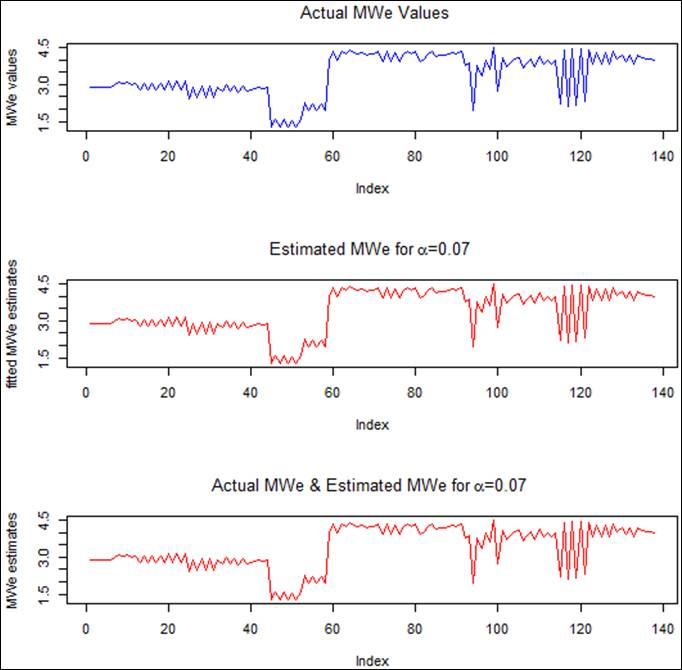Figure 7. Graph showing the fit of actual megawatts, estimated megawatts and a comparison between the two with α= 0.07, λ= 2 and assuming Gaussian errors.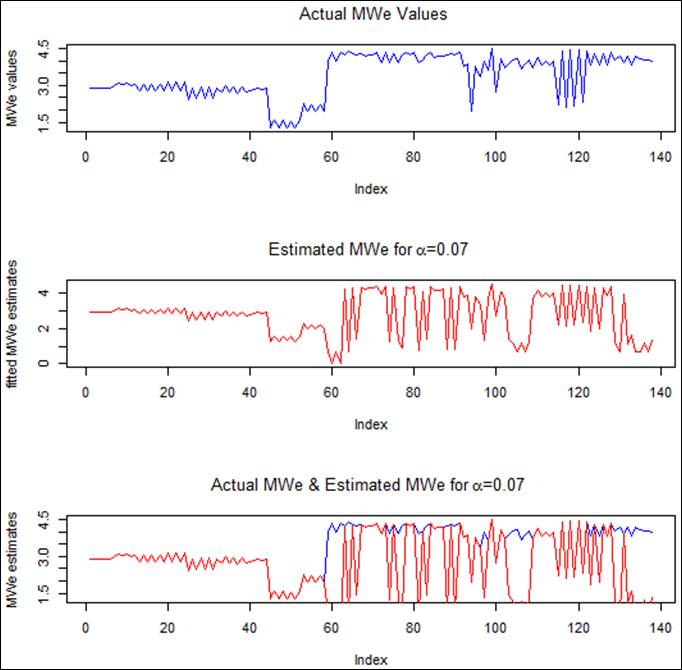Figure 8. Graph showing the fit of actual megawatts, estimated megawatts and a comparison between the two with α= 0.07, λ = 2 and assuming symmetric errors.

3.4. Fitting of the Loess Model to Other Wells

A sample of two other wells was taken where the adopted model was fitted. From the findings in Figure 9 & 10 below, it is evident that loess with a smoothing parameter of 0.07 and polynomial of order 2 is able to model a geothermal well data of well MW-01.Figure 9. Graph showing the fit of actual megawatts, estimated megawatts and a comparison between the two with α= 0.07 and λ= 2 for well MW-10A.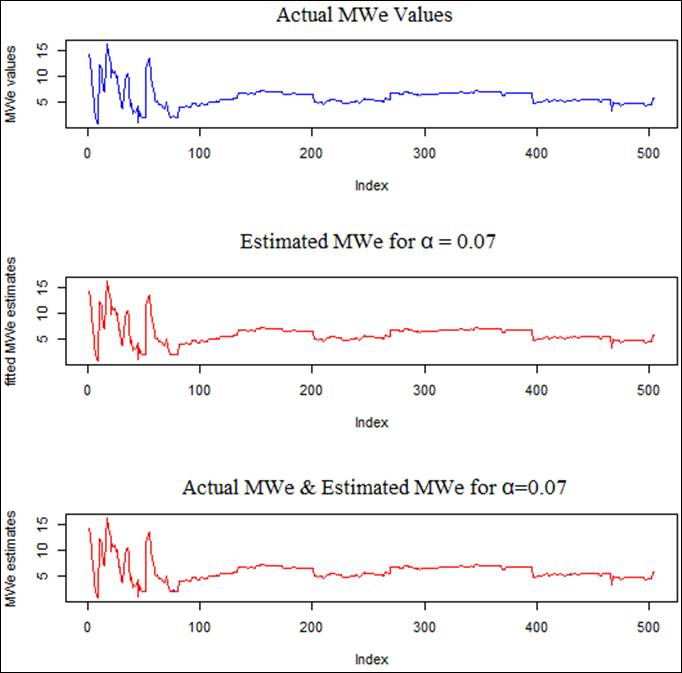Figure 10. Graph showing the fit of actual megawatts, estimated megawatts and a comparison between the two with α= 0.07 and λ= 2 for well MW-19.

3.5. Comparison with OLS

Comparison between the loess estimates and OLS shows that the loess estimate with α=0.07 produced a lower standard error (1.520978e-07) compared to the OLS estimates (0.06029956). These results show that the loess estimates produce better estimates of predicted values.

From Figure 11 it is seen that while the actual values of MWe fitted perfectly with the estimated values for smoothing parameter of 0.07, on the other hand the estimated values for the OLS deviated so much from the actual values as shown by the green lines. While it's easy to see the difference between the actual MWe values and OLS estimates, it is not easy to distinguish the actual MWe values with those obtained by the smoothing function since the two graphs fit exactly to one another. It can be concluded that the loess method performs better than the OLS method.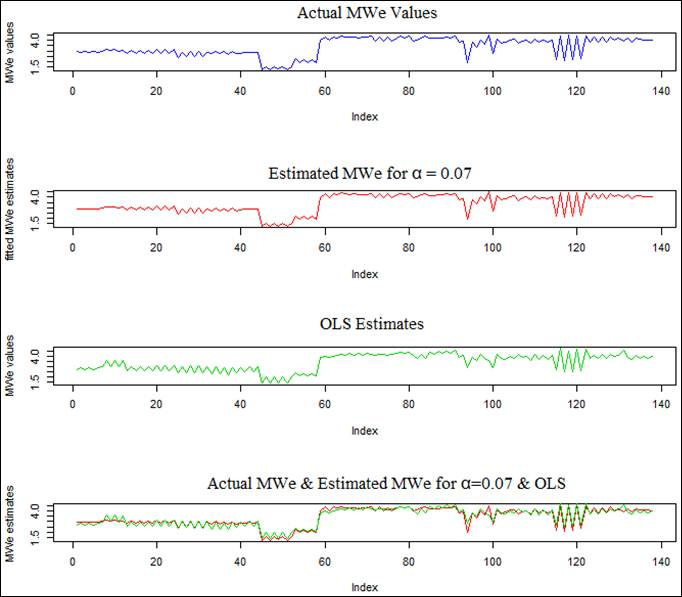Figure 11. Graph showing the fit of actual megawatts, estimated megawatts with α= 0.07, λ = 2, OLS estimates and a comparison between the two methods.

4. Conclusions

Locally weighted regression also known as loess which is non-parametric was used to fit the data. Robust parametric methods could also be used but this required that the functional form of the relationship between predictor and response variables should be known in advance; with this we could not apply the parametric methods since the functional form of the data was not known.

The order of polynomial that fits the data is 2. The assumption that polynomial of order 1 fits almost all the cases was disapproved. Polynomial of degree 0 could also not fit the data.

Locally weighted regression (loess) with a bandwidth (smoothing parameter) of 0.07 and a polynomial of degree 2 was able to fit the geothermal well output data. Empirical studies on other wells were also performed and the results were the same.

The errors of the fitted values assume a Gaussian distribution and therefore locally weighted regression was used to fit the data. Further research done to compare locally weighted regression method and the ordinary least squares method shows that the later does not fit the data.

Recommendation

Geothermal well discharge data is characterized by outliers which may affect the ultimate determination of amount of a well output. It is therefore necessary that before application of commonly used Russel James method, further statistical data processing to remove the errors should be carried out before being used as the input to the Russel James method.

References

1. Cleveland W. S., 1979. Robust Locally Weighted Regression and Smoothing Scatterplots. Journal of the American Statistical Association, 74: 829 – 836.
2. Cleveland W. S. and Devlin S. J., 1988. Locally weighted regression: An approach to regression analysis by local fitting. Journal of the American Statistical Association, 83: 596-610.
3. Cleveland W. S., and Loader C. L., 1996. Smoothing by local regression: principles and methods. In Hardle W. and Schimek M. G., editors, Statistical Theory and Computational Aspects of Smoothing, pages 10-49. Springer, New York.
4. Grant M. A., Donaldson I. G. and Bixley P. F., 1982: Geothermal reservoir engineering. Academic Press Ltd., New York, 369.
5. Heya M. M., 2002. Geothermal exploration and development in Kenya. Ministry of Energy, Kenya.
6. Houssein D. E., 2008. Geothermal resource assessment through well testing and production response modelling, United Nations University, MSC thesis.
7. Jacoby W. G., 2000. Loess: a non-parametric, graphical tool for depicting relationships between ariables, Department of Government & International Studies, University of South Carolina, Columbia, USA.
8. James R., 1962. Steam water critical flow through pipes, Proceedings of the Institution of Mecanical engineers, London 176-26, 739-748.
9. Lagat J., Mbia P. and Muturia C. L., 2010: Menengai prospect: Investigations for its geothermal potential. Contributions from: Njue L., Mutonga M., Malimo S., Kanda I.
10. Mungania J., 2004. Geological studies of Menengai geothermal prospect, Kenya Electricity Generating Company Ltd., internal report, 18.
11. Ofwona C. O., Kipyego E. K. and Suwai J. J., 2011. Preliminary well test data of Menengai exploration wells, Kenya Geothermal Conference Paper.
12. Suwai J. J., 2011. Preliminary reservoir analysis of Menengai geothermal field exploration wells, Geothermal Training Programme, Reporting.

 Contents 1. 2. 2.1. 2.2. 2.3. 3. 3.1. 3.2. 3.3. 3.4. 3.5. 4.
Article ToolsAbstractPDF(2965K)